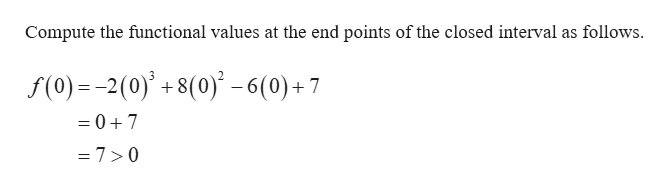Find the smallest integer a such that the Intermediate Value Theorem guarantees that f(x) has a zero on the interval [0,a].f(x)=−2x^3+8x^2−6x+7

Question

Find the smallest integer a such that the Intermediate Value Theorem guarantees that f(x) has a zero on the interval [0,a].

f(x)=−2x^3+8x^2−6x+7
Step 1

Consider the given function and interval.

Consider the function as f(x)=2x3 + 8x2− 6x+7 and interval [0, a].

The give polynomial function is continuous over the closed interval [0, a].

Intermediate Value Theorem:

Let f be a continuous function on the closed interval [a, b], then if ...help_outlineImage TranscriptioncloseCompute the functional values at the end points of the closed interval as follows. f(0) 2(0)+8(0)-6(0)+7 = 07 =7>0 fullscreen

Want to see the full answer?

See Solution

Want to see this answer and more?

Our solutions are written by experts, many with advanced degrees, and available 24/7

See Solution
Tagged in

Other